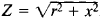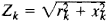# Electrical Impedance

(redirected from Complex electric impedance)

## Electrical impedance

The measure of the opposition that an electrical circuit presents to the passage of a current when a voltage is applied. In quantitative terms, it is the complex ratio of the voltage to the current in an alternating current (ac) circuit.

A generalized ac circuit may be composed of the interconnection of various types of circuit elements. The impedance of the circuit is given by Z = V/I, where Z is a complex number given by Z = R + jX. R, the real part of the impedance, is the resistance of the circuit, and X, the imaginary part of the impedance, is the reactance of the circuit. The units of impedance are ohms. See Electrical resistance, Reactance

## Impedance, Electrical

a quantity characterizing the opposition presented by a circuit to an alternating current. Often called simply impedance, it is measured in ohms. In the case of a sinusoidal alternating current, the impedance is expressed by the ratio of the amplitude of the voltage applied to the circuit terminals and the amplitude of the current throught the circuit; here, the impedance is equal to, where r is the resistance and x is the reactance. In the case of a nonsinusoidal alternating current, the impedance is determined separately for each kth har monic component:.

## electrical impedance

[i′lek·trə·kəl im′pēd·əns]
Also known as impedance.
(electricity)
The total opposition that a circuit presents to an alternating current, equal to the complex ratio of the voltage to the current in complex notation. Also known as complex impedance.
The ratio of the maximum voltage in an alternating-current circuit to the maximum current; equal to the magnitude of the quantity in the first definition.
Site: Follow: Share:
Open / Close